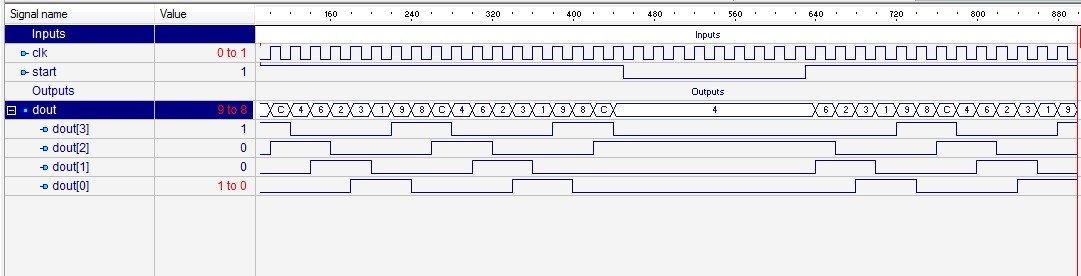Saturday, 27 July 2013

Design of Stepper Motor Driver (Half Step) using Behavior Modeling Style (VHDL Code).

Design of Stepper Motor Driver (half step) using Behavior Modeling Style -Output Waveform :   Stepper Motor Driver (Half Step).

VHDL Code -

-------------------------------------------------------------------------------
--
-- Title       : stepper_driver_half_step
-- Design      : vhdl_upload2
-- Author      : Naresh Singh Dobal
-- Company     : nsdobal@gmail.com
-- VHDL Programs &  Exercise with Naresh Singh Dobal.
--
-------------------------------------------------------------------------------
--
-- File        : Stepper Motor Driver (half_step).vhd

library IEEE;
use IEEE.STD_LOGIC_1164.all;
use ieee.std_logic_arith.all;
use ieee.std_logic_unsigned.all;

entity stepper_driver_half_step is
port(
clk : in STD_LOGIC;
start : in STD_LOGIC;
dout : out STD_LOGIC_VECTOR(3 downto 0)
);
end stepper_driver_half_step;

architecture stepper_driver_arc of stepper_driver_half_step is
begin

stepper_driver : process (clk,start) is
variable m : std_logic_vector (2 downto 0) := "000";
begin
if (start='1') then
if (rising_edge (clk)) then
m := m + 1;
end if;
end if;

case m is
when "000" => dout <= "1000";
when "001" => dout <= "1100";
when "010" => dout <= "0100";
when "011" => dout <= "0110";
when "100" => dout <= "0010";
when "101" => dout <= "0011";
when "110" => dout <= "0001";
when others => dout <= "1001";
end case;
end process stepper_driver;

end stepper_driver_arc;